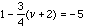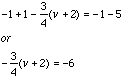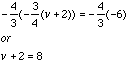Beth high school 9th grade student 1-3/4(v+2)=-5 Hi Beth, If you want to solve the equationfor v then you need to work through a sequence of steps to end with the equation v = ??? At each step you need to perform identical operation to each side of the equation to maintain the equality. The first step is to add -1 to both sides of the equation to getNow multiply both sides by -4/3Finally add -2 to both sides to arrive at the solution v = 6 Cheers, Penny Go to Math Central